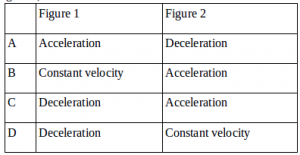# Free fall motion – problems and solutions

1. A stone free fall from the height of 45 meters. If the acceleration due to gravity is 10 ms-2, what is the speed of the stone when hits the ground?

Known :

Height (h) = 45 meters

Acceleration due to gravity (g) = 10 m/s2

Wanted : The final velocity of the stone when it hits the ground (vt)

Solution :

The equation of free fall motion :

vt2 = 2 g h

The final velocity of the stone :

vt2 = 2 (10)(45) = 900

vt = √900 = 30 m/s2

2. An object free fall from a height without the initial velocity. The object hits the ground 2 seconds later. Acceleration due to gravity is 10 ms-2. Determine height

Known :

Time interval (t) = 2 seconds

Acceleration due to gravity (g) = 10 m/s2

Wanted : Height (h)

Solution :

The equation of the free fall motion :

h = ½ g t2

Height :

h = ½ (10)(2)2 = (5)(4) = 20 meters

[irp]

3.A 2-kg object free fall from a height of 20 meters above the ground. What is the time interval the object in air ? Acceleration due to gravity is 10 ms-2

Known :

Height (h) = 20 meters

Acceleration due to gravity (g) = 10 m/s2

Wanted : Time interval (t)

Solution :

The equation of free fall motion :

h = ½ g t2

Time interval :

20 = ½ (10)(t2)

20 = (5)(t2)

20/5 = t2

4 = t2

t = √4

t = 2 seconds

4. Two objects, object 1 and object 2, are free fall from a height of h1 and h2 at the same time. If h1 : h2 = 2: 1, what is the ratio of the time interval of the object 1 to the object 2.

Known :

The height of the object 1 (h1) = 2

The height of the object 2 (h2) = 1

Acceleration due to gravity = g

Wanted : t1 : t2

Solution :

Object 1 :

h1 = 1/2 g t12

2 = 1/2 g t12

(2)(2) = g t12

4 = g t12

4/g = t12

t1 = √4/g

Object 2 :

h2 = 1/2 g t22

1 = 1/2 g t22

(2)(1) = g t22

2 = g t22

2/g = t22

t2 = √2/g

The ratio of the time interval :

t1 : t2

4/g : √2/g

(√4/g)2 : (√2/g)2

4/g : 2/g

4 : 2

2 : 1

[irp]

5. An object dropped from a height of h above the ground. The final velocity when object hits the ground is 10 m/s. What is the time interval to reach ½ h above the ground. Acceleration due to gravity is 10 m/s2.

Known :

The final velocity (vt) = 10 m/s

Acceleration due to gravity (g) = 10 m/s2

Wanted : The time interval to reach 1/2 h above the ground

Solution :

The height of h :

vt2 = 2 g h

102 = 2 (10) h

100 = 20 h

h = 100 / 20

h = 5 meters

The height of 1/2 h = 1/2 (5 meters) = 2.5 meters. The time interval needed to reach 2.5 meters above the ground :

h = 1/2 g t2

2.5 = 1/2 (10) t2

2.5 = 5 t2

t2 = 2.5 / 5 = 0.5 = (0.25)(2)

t = √(0.25)(2) = 0.5√2 = 1/2 √2 seconds

6.The free fall motion of coconut (figure 1) and the motion of a ball thrown vertically upward to the highest point by a student (figure 2). Determine the kind of both motions.Solution :

Figure 1 = free fall motion = Acceleration

Figure 2 = vertical motion = Deceleration

[irp]

7. A stone free fall from a building. The time interval needed by a stone to reach the ground is 3 seconds and acceleration due to gravity is 10 m/s2. Determine the height of the building.

A. 15 m

B. 20 m

C. 30 m

D. 45 m

Known :

Time interval (t) = 3 seconds

Acceleration due to gravity (g) = 10 m.s-2

Wanted: Height of building (h)

Solution :

Known: time interval (t) and acceleration due to gravity (g), wanted: height (h) so use the equation of free fall motion: h = ½ g t2

h = ½ (10)(3)

h = (5)(3)

h = 15 metes

8. A fruit free fall from its tree at the height of 12 m above the ground. If acceleration due to gravity is g = 10 m/s2 and the friction of air ignored, then determine the height of the fruit above the ground after 1 second.

A. 7 m

B. 6 m

C. 5 m

D. 4 m

Known :

Height of tree (h) = 12 meters

Acceleration due to gravity (g) = 10 m/s2

Time interval (t) = 1 second

Wanted: The height of the fruit above the ground

Solution :

After 1 second, fruit free fall as far as :

h = ½ g t2 = ½ (10)(1)2 = (5)(1) = 5 meters

The height of the fruit above the ground after 1 second :

12 meters – 5 meters = 7 meters